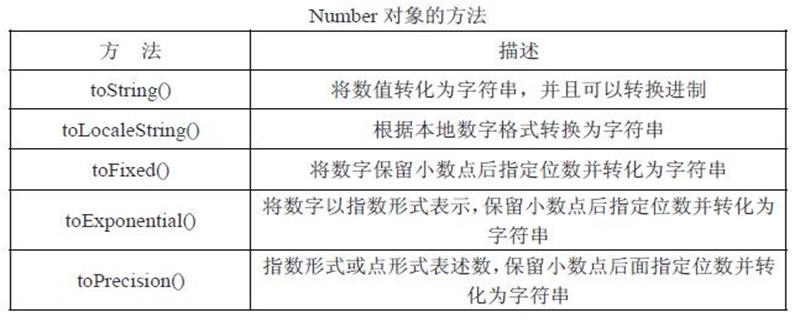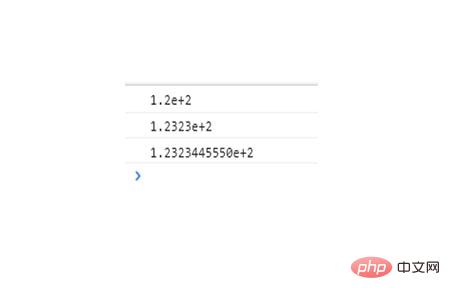# JS中格式化数字有哪几种方法

js中格式化数字的方法有：toFixed() 方法将数字转换为指定小数位数的数字、toPrecision() 方法在超出指定位数时转换为指数计数法、toExponential() 方法把值转换为指数计数法

【推荐课程：JavaScript教程toFixed() 方法

`Number.toFixed(num)`

num：指小数的位数，它的取值范围是 0 ~ 20 之间的值，包括 0 和 20，如果不写这个参数的话将会用0来代替。

```<script>
var number=123.2344555
console.log(number.toFixed(0));
console.log(number.toFixed(4));
console.log(number.toFixed(10));
</script>```toPrecision() 方法

`Number.toPrecision(num)`

num：指必须被转换为指数计数法的最小位数。它的取值范围是 1 ~ 21 之间（且包括 1 和 21），如果不写这个参数的话，则会调用方法 toString()，而不是把数字转换成十进制的值。

```<script>
var number=123.2344555
console.log(number.toPrecision(1));
console.log(number.toPrecision(4));
console.log(number.toPrecision(10));
</script>```toExponential() 方法

`Number.toExponential(num)`

num：指指数计数法中的小数位数，是 0 ~ 20 之间的值（包括 0 和 20），如果不写这个参数，将会使用尽可能多的数字。

```<script>
var number=123.2344555
console.log(number.toExponential(1));
console.log(number.toExponential(4));
console.log(number.toExponential(10));
</script>```#### 相关文章推荐

• JS格式化数字保留两位小数点示例代码_javascript技巧 • JavaScript格式化数字的函数代码_javascript技巧 • JS格式化数字

• 取消 发布评论 发送